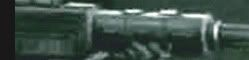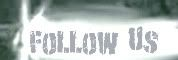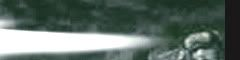## Friday, 26 November 2010

### Calculating probabilities in Warhammer using binomial distribution

I want to get a bit technical with this post. I know the title sounds somewhat scary but if I can get my head round to it then everybody else can too! I want to show you the correct way of calculating the probability of a repeated event in  Warhammer (e.g. what's the probability of killing a Tactical Space Marine with 3 meltagun shots at BS3 if he is not getting a cover save) and will show you a handy tool so that you can make your own calculations.

Calculating a single event is very easy to do. Lets say that we want so see what are the chances of a Guardsman with a meltagun gunning down a Space Marine that is out of cover. All we have to do is multiply his chance to hit (1/2 since he has BS3) to his chance to wound (5/6 since it is S8 vs T4) to his armour save (in this case since the weapon is AP1 and the SM has a save of +3 he isn't getting any). Simple enough.  In our case the result would be 1/2 x 5/6 = 5/12 almost 0,42 which is 42%. Now, this is the elementary part.

The hard thing is to find the probability of multiple meltagun shots killing the SM. Do we simply multiply the number of shots with the probability of a single successful shot ? Let's see : 3 x 0,42 = 1,26. But what does this even mean? That we are 126% possible that he is going to die? That doesn't even make sense. In reality this number gives us many probabilities at the same time (probability of one shot killing him + both shots killing him + probability of all three shots killing him) that is why it's above 100%. But that's not what we want to calculate. We need distinct results for each probability we know what's going on. The correct way to do this is by using binomial distribution.

But what is this binomial distribution? It is simply a way to measure our chance of succeeding in a binomial experiment presented in a certain distribution form. Now, most events in Warhammer that involve killing something can be considered a binomial experiment so this applies well to our case. Using the example I gave above I will explain why.

There are a few requirements for a binomial experiment. First of all it has to lead in only two separate results (the SM will either live or die, nothing else in between so it's either 0 or 1).  Then the experiment must be repeated a few times (3 meltagun shots). Furthermore the probability of success must remain the same through all trials (each meltagun shot has 42% of killing him constantly). Lastly all the trials must be completely independent from each other which is true again in our case because one does not affect the other.

So we need a function that takes all this into accounts and gives us the result. The problem is that these equations are very complicated. You can take a look here but it's disheartening to say the least. But have no fear. Some very nice people have created a calculator for us folks who don't own a PhD in maths. Check it out here:

Even though we have a calculator we need to know what we need to input and how to translate the raw data we get back:

• Probability of success on a single trial: What we calculated in the example above. In our case it's 0.42
• Number of trials: How many times the event will happen. In our case is three meltagun shots so 3.
• Number of successes (x): How many times we want to kill something. In our case is a single SM so we will input  1 but if there is more we can change the number accordingly.

OK, now hit the "Calculate" button and lets analyse the data:

• Binomial Probability: P(X = 1): 0.423864 This number shows us the probability of killing the SM exactly once.
• Cumulative Probability: P(X < 1): 0.195112 This number shows us the probability of the SM surviving.
• Cumulative Probability: P(X < 1): 0.618976 This shows us the probability of the SM surviving or dying once.
• Cumulative Probability: P(X > 1): 0.381024 This is the probability of the SM dying more than once.
• Cumulative Probability: P(X > 1): 0.804888 And this is what we are mostly interested in. It shows us the chance of the SM dying at least once or more. In simple terms this is the total chance of the Space Marine dying (we don't care if he dies more than once since the result is the same).

This is an extremely handy tool for all the mathhammer aficionados out there. Just calculate the probability of something you want to know and use this amazing tool and get the probabilities of multiple shots, attacks etc. Hope this is helpful and easy to understand. If you have any questions or you would like me to elaborate some more on the subject please let me know.

1.That's a nice bit of kit. Shame it doesn't work out the Confidence Interval too ;-)

2.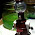3.@ C&F: That's what beer is for, mate :P

4.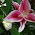Wow. I totally avoid mathhammer for many reasons, but if I ever want to do it, I'll steal this little tool.

5.Turn to the dark side Loquacious, use mathhammer and IG! The dark side is powerful!

By the way I would like to thank Messanger of Death for the inclusion of this article in Imperial Life + Happy fiftieth post to me!

6.7.Hey, thanks. I don't know how awesome mathhammer is but it sure is a useful too :)

8."The hard thing is to find the probability of multiple meltagun shots killing the SM. Do we simply multiply the number of shots with the probability of a single successful shot ? Let's see : 3 x 0,42 = 1,26. But what does this even mean? That we are 126% possible that he is going to die? That doesn't even make sense. In reality this number gives us many probabilities at the same time (probability of one shot killing him + both shots killing him + probability of all three shots killing him) that is why it's above 100%."

Actually this number 1.26 has a meaning : It's the expected value, or the average number of kills for 3 melta guards firing at 3 Space Marines.